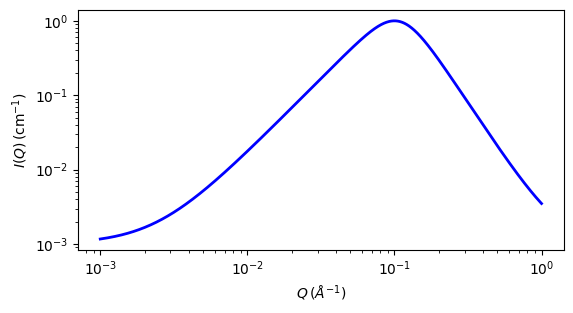# spinodal

Spinodal decomposition model

Parameter

Description

Units

Default value

scale

Scale factor or Volume fraction

None

1

background

Source background

cm-1

0.001

gamma

Exponent

None

3

q_0

Correlation peak position

-1

0.1

The returned value is scaled to units of cm-1 sr-1, absolute scale.

Definition

This model calculates the SAS signal of a phase separating system undergoing spinodal decomposition. The scattering intensity $$I(q)$$ is calculated as

$I(q) = I_{max}\frac{(1+\gamma/2)x^2}{\gamma/2+x^{2+\gamma}}+B$

where $$x=q/q_0$$, $$q_0$$ is the peak position, $$I_{max}$$ is the intensity at $$q_0$$ (parameterised as the $$scale$$ parameter), and $$B$$ is a flat background. The spinodal wavelength, $$\Lambda$$, is given by $$2\pi/q_0$$.

The definition of $$I_{max}$$ in the literature varies. Hashimoto et al (1991) define it as

$I_{max} = \Lambda^3\Delta\rho^2$

whereas Meier & Strobl (1987) give

$I_{max} = V_z\Delta\rho^2$

where $$V_z$$ is the volume per monomer unit.

The exponent $$\gamma$$ is equal to $$d+1$$ for off-critical concentration mixtures (smooth interfaces) and $$2d$$ for critical concentration mixtures (entangled interfaces), where $$d$$ is the dimensionality (ie, 1, 2, 3) of the system. Thus 2 <= $$\gamma$$ <= 6. A transition from $$\gamma=d+1$$ to $$\gamma=2d$$ is expected near the percolation threshold.

As this function tends to zero as $$q$$ tends to zero, in practice it may be necessary to combine it with another function describing the low-angle scattering, or to simply omit the low-angle scattering from the fit.Fig. 119 1D plot corresponding to the default parameters of the model.

Source

spinodal.py

References

1. H. Furukawa. Dynamics-scaling theory for phase-separating unmixing mixtures: Growth rates of droplets and scaling properties of autocorrelation functions. Physica A 123, 497 (1984).

2. H. Meier & G. Strobl. Small-Angle X-ray Scattering Study of Spinodal Decomposition in Polystyrene/Poly(styrene-co-bromostyrene) Blends. Macromolecules 20, 649-654 (1987).

3. T. Hashimoto, M. Takenaka & H. Jinnai. Scattering Studies of Self-Assembling Processes of Polymer Blends in Spinodal Decomposition. J. Appl. Cryst. 24, 457-466 (1991).

Authorship and Verification

• Author: Dirk Honecker Date: Oct 7, 2016

• Last Modified by: Steve King Date: Oct 25, 2018

• Last Reviewed by: Steve King Date: Oct 25, 2018